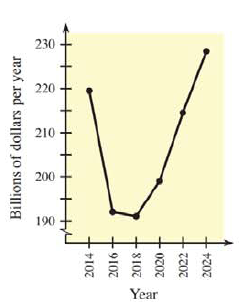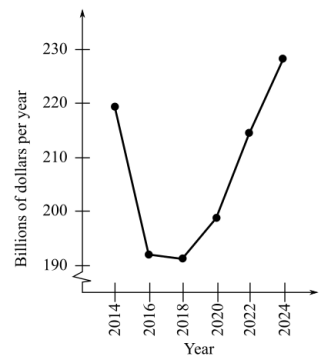Chapter 13.1, Problem 34E### Mathematical Applications for the ...

11th Edition
Ronald J. Harshbarger + 1 other
ISBN: 9781305108042

#### Solutions

Chapter
Section### Mathematical Applications for the ...

11th Edition
Ronald J. Harshbarger + 1 other
ISBN: 9781305108042
Textbook Problem

# Oil imports Crude oil and petroleum products are imported continuously by the United States. The following table and figure show the net expenditures for U.S. oil imports for selected years (in billions of dollars per year) (Source: Energy Information Administration), (a) Use n = 5 equal subdivisions and left-hand end-points to estimate the area under the graph from 2014 to 2024.(b) What does this area represent in terms of U.S. oil imports? Year Billions of Dollars 2014 219.5 2016 192.0 2018 191.0 2020 198.9 2022 214.5 2024 228.4(a)

To determine

To calculate: The area under the curve in the graph using n=5 equal subdivisions and left-hand endpoints from 2014 to 2024 where the graph shows the net expenditures for U.S. oil imports.Explanation

Given Information:

The provided graph and table are,

 Years Billions Of Dollars 2014 219.5 2016 192 2018 191 2020 198.9 2022 214.5 2024 228.4

Formula used:

The base of the rectangles to approximate the area is ban where the interval [a,b] on which function is defined is divided into n subintervals.

The height of the rectangles is the value of the function calculated at the left-hand end point of the interval containing the base.

The area of a rectangle is base×height.

The approximated area under the curve is sum of the areas of each rectangle.

Calculation:

Consider the provided table and graph,

 Years Billions Of Dollars 2014 219.5 2016 192 2018 191 2020 198.9 2022 214.5 2024 228.4

Since, the table represents the corresponding values of y at various x.

Thus, the height of the function from 2014 to 2024 is 219.5, 192, 191, 198.9 and 214.5 respectively.

The base of the rectangles to approximate the area is ban where the interval [a,b] on which function is defined is divided into n subintervals

(b)

To determine

What the area under the curve in the graph represents where the graph shows the net expenditures for U.S. oil imports.### Still sussing out bartleby?

Check out a sample textbook solution.

See a sample solution

#### The Solution to Your Study Problems

Bartleby provides explanations to thousands of textbook problems written by our experts, many with advanced degrees!

Get Started

#### Find more solutions based on key concepts